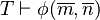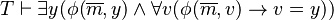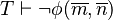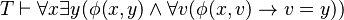Difference between revisions of "User:IssaRice/Computability and logic/Expresses versus captures"

The expresses versus captures distinction is an important one in mathematical logic, but unfortunately the terminology differs wildly between different texts. The following table gives a comparison.

• Expressing is done by a language. There is only one form of expressing; I think this follows from the wikipedia:Law of excluded middle.
• Capturing is done by a theory or by axioms. There are two forms of capturing: strong capture (corresponding to deciding), and weak capture (corresponding to recognizing, or semi-deciding).

Capturing functions

For functions, it seems like there are at least four different strengths.

1.$f$ is captured by$\phi(x,y)$ iff for all$m,n$ (i) if$f(m) = n$ then$T \vdash \phi(\overline{m}, \overline{n})$ and (ii)$T \vdash \exists y (\phi(\overline{m}, y) \wedge \forall v(\phi(\overline{m}, v) \to v=y))$.
2.$f$ is captured by$\phi(x,y)$ iff for all$m,n$, if$f(m) = n$, then$T \vdash \forall y (\phi(\overline m,y) \leftrightarrow y = \overline n)$.
3.$f$ is captured by$\phi(x,y)$ iff for all$m,n$ (i) if$f(m)=n$ then$T \vdash \phi(\overline m, \overline n)$, and (ii) if$f(m)\ne n$ then$T \vdash \neg \phi(\overline m, \overline n)$.
4.$f$ is captured by$\phi(x,y)$ iff (i) for all$m,n$, if$f(m) = n$ then$T \vdash \phi(\overline m, \overline n)$, and (ii) we have$T \vdash \forall x \exists y (\phi(x,y) \wedge \forall v (\phi(x,v) \to v=y))$.
5.$f$ is captured by$\phi(x,y)$ iff for all$m,n$ (i) if$f(m)=n$ then$T \vdash \phi(\overline m, \overline n)$, and (ii) if$f(m)\ne n$ then$T \nvdash \phi(\overline m, \overline n)$.

Comparison of usage patterns

Text "Expresses" "Captures"
Peter Smith. Godel book (see especially footnote 9 on p. 45) expresses captures
Leary & Kristiansen defines represents
Goldrei defines (but the book also uses "represents")
Boolos, Burgess, Jeffrey (5th ed) arithmetically defines defines (for sets), represents (for functions)
Wikipedia arithmetically defines, i think this page uses "defines" in the expresses sense this page uses "represents", but I don't think there's a standalone article for the concept